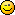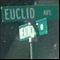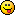# Math Is Fun Forum

Discussion about math, puzzles, games and fun.   Useful symbols: ÷ × ½ √ ∞ ≠ ≤ ≥ ≈ ⇒ ± ∈ Δ θ ∴ ∑ ∫  π  -¹ ² ³ °

You are not logged in.

## #1 2008-08-15 09:54:54

JaneFairfax
MemberRegistered: 2007-02-23
Posts: 6,868

### Algebraic topology

I will summarize the results I developed and presented another thread in a more coherent and easier-to-follow fashion here.1. HOMOTOPY

You have two topological spaces X and Y and two continuous functions

. Suppose for each
, there corresponds a continuous function
such that
and
for all
.

A continuous function

is callled a homotopy from f to g. We say that f and g are homotopic iff there exists a homotopy from f to g, and we write
; we can also express the fact that H is a homotopy from f to g by writing
.

The relation is homotopic to is an equivalence relation on

, the class of all continuous functions from X to Y.

(i) For any

, the function
is a homotopy from f to itself.

(ii) For any

, if
, then
is a homotopy from g to f.

(ii) For any

, if
and
, define
as follows.

Then

.

the equivalence classes under this equivalence relation are called homotopy classes.

Offline

## #2 2008-08-24 04:52:49

JaneFairfax
MemberRegistered: 2007-02-23
Posts: 6,868

### Re: Algebraic topology

2. RELATIVE HOMOTOPY

Let X, Y be topological spaces,

, and A a subset of X such that
for all
. If H is a homotopy from f to g such that
for all
, H is called a homotopy relative to A (or homotopy rel A) from f to g, and we can write
.

Like ordinary homotopy, relative homotopy is an equivalence relation on

. Indeed, if
, H is just an ordinary homotopy.

Offline

## #3 2008-09-14 04:41:32

JaneFairfax
MemberRegistered: 2007-02-23
Posts: 6,868

### Re: Algebraic topology

3. PATHS

Let X be a topological space and

. A path in X from a to b is a continuous function
with
and
.

If p is a path in X from a to b and q is a path in X from b to c, the product path

is the path from a to c defined by

Offline

## #4 2008-09-14 05:11:45

LQ
Real Member
Registered: 2006-12-04
Posts: 1,285

### Re: Algebraic topology

I'm amazed, sounds important

I see clearly now, the universe have the black dots, Thus I am on my way of inventing this remedy...

Offline

## #5 2008-09-14 05:32:17

Ricky
ModeratorRegistered: 2005-12-04
Posts: 3,791

### Re: Algebraic topology

In differential geometry, you also define the set of paths to a point to be the tangent space at that point.  The paths are of the form:

Such that

Where M is your manifold.  We then use the tangent space to define what a "derivative" means.

"In the real world, this would be a problem.  But in mathematics, we can just define a place where this problem doesn't exist.  So we'll go ahead and do that now..."

Offline

## #6 2008-09-14 05:46:22

JaneFairfax
MemberRegistered: 2007-02-23
Posts: 6,868

### Re: Algebraic topology

Thanks Ricky.Later, when I come to n-dimensional homotopy groups, I will be defining n-dimensional paths as continuous functions from the n-dimensional hypercube to X  but for the time being I avoid jumping the gun.Offline

## #7 2016-04-20 11:09:01

Nehushtan
MemberRegistered: 2013-03-09
Posts: 957

### Re: Algebraic topology

4. BORSUK–ULAM THEOREM

Offline

## #8 2016-04-21 00:18:07

Nehushtan
MemberRegistered: 2013-03-09
Posts: 957

### Re: Algebraic topology

5. HAM-SANDWICH THEOREM

It is always possible to slice a three-layered ham sandwich with a single cut of a knife in such a way that each layer of the sandwich is divided into two exactly equal halves by the cut.

The ham-sandwich theorem can be proved using the Borsuk–Ulam theorem.

Last edited by Nehushtan (2016-04-21 00:30:28)